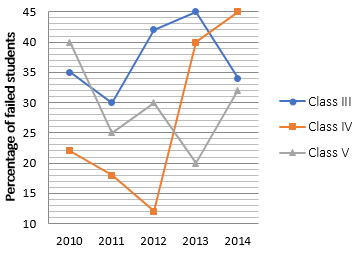### SBI PO Quantitative Aptitude Quiz

Here, we are providing free crash course for SBI PO as there is left not enough time to deal in details. The questions asked in the quantitative aptitude section are calculative and very time-consuming. But once dealt with proper strategy, speed, and accuracy, this section can get you the maximum marks in the examination. Following is the Quantitative Aptitude quiz to help you practice with the best of latest pattern questions

Directions (1-5): The following graph shows the percentage of failed students in three different classes of a school in five different years.
Study the graph carefully to answer the questions based.
Note: Total no. of students in each class in each year is not same unless it is said.Q1. In 2012, in class V, 140 students passed which is equal to 175% of failed students of same class in 2013. Find the ratio of failed students in 2012 of class V and passed students in 2013 of class V.

5 : 16
3 : 16
4 : 17
3 : 17
None of these

Q2. If the total strength of students in 2010 in class IV was 150 and it increases every year by 50, then find the number of passed students of class IV in 2013.
182
160
180
170
None of these

Q3. The ratio of girls to boys who failed in class III in 2014 was 7 : 10. One-seventh i.e. 5 of these girls passed when their answer sheet were re-evaluated which made the number of passed girls i.e. 84 in 2014, which is 20% more than those in 2010 in same class. Find the number of passed boys in 2010 in class III. Total students in class III in 2014 and 2010 are in ratio 5 : 4.
60
65
70
62
None of these

Q4. In 2012, 105 students failed in class III, while 198 students passed in class IV. The number of passed boys is 17 more than the passed girls in class III and the number of failed girls is 15 less than the failed boys in class IV. Find the difference in failed boys of class IV and passed girls of class III.
45
50
52
43
None of these

Q5. Find the average of passed students of class IV throughout the given years, if number of students in class IV is same in all the given years.
76.2%
70.8%
72.6%
70.2%
None of these

Directions (6-10): Find the missing term in the following number series.

Q6. 729, 81, ?, 27, 81, 9

27
243
233
241
162

Q7. 1, 1, 2, 6, 24, 120, 720, ?
4050
5060
5040
6050
4455

Q8. 0, 1, 4, 9, 16, 25, ?
49
64
81
36
32

Q9. 1296, 1444, 1600, 1764, ?
1936
1986
1996
1946
1846

Q10. 1728, 1331, 1000, 729, ?
1024
512
128
216
343

Q11. If the marked price of a TV is Rs. 12,600 and a person buys it at a discount of 100/3%. after using it for 6 months, he spends Rs. 2,400 on its maintenance, then at what price the person should sell the TV to make a profit of 50/3%?
Rs. 9,600
Rs. 10,800
Rs. 12,600
Rs. 16,900
Rs. 12,800

Q12. Ravi, Jyoti and Shushma enter into a partnership business. Ravi invested 7/4th of the sum invested by Jyoti and Shushma invested 4/5th of the sum invested by Ravi, who also manages the business and gets extra 50/3% of total profit for it, and remaining profit is distributed in the ratio of their investments among them. If profit share of Jyoti’s after one year is Rs. 8000, then what is the total profit share of Ravi?
Rs. 24,640
Rs. 20,460
Rs. 16,640
Rs. 20,640
Rs. 18,640

Q13. What is the probability of making a five digits number which is divisible by 5 by using digits out of 0, 1, 2, 4, 5, 6, 8, 9, without repeating any digit.
31/49
41/49
22/49
17/49
13/49

Q14. Quantity 1: Share of Ghanshyam. Ram, Shyam and Ghanshyam working alone can complete a work in 12 days, 18 days and 24 days respectively. All of them start working together and after working 2 days, Ram left the work. After next 4 days Shyam also left the work. Rest of the work is done by Ghanshyam. They got Rs. 2880 for whole of the work.
Quantity 2: Difference between the share of Prabhat and Sandeep. A sum of Rs 72500 is divided among Abhishek, Sandeep and Prabhat. Abhishek receives 10% more than Prabhat, who receives 25 % more than Sandeep.

Quantity 1 > Quantity 2
Quantity 1 ≥ Quantity 2
Quantity 1 ≤ Quantity 2
Quantity 1 < Quantity 2
Quantity 1 = Quantity 2 or no relation.

Q15. Ratio of Volume of sphere to Volume of Cylinder is 2 : 3. Curved surface area of sphere is 616 cm². Height of Cylinder is double of its radius.
Quantity 1 – Volume of cube whose Side is equal to height of Cylinder.
Quantity 2 – Volume of cone whose radius is twice of radius of sphere and height is 15 cm.

Quantity 1 > Quantity 2
Quantity 1 ≥ Quantity 2
Quantity 1 ≤ Quantity 2
Quantity 1 < Quantity 2
Quantity 1 = Quantity 2 or no relation.

You May also like to Read: